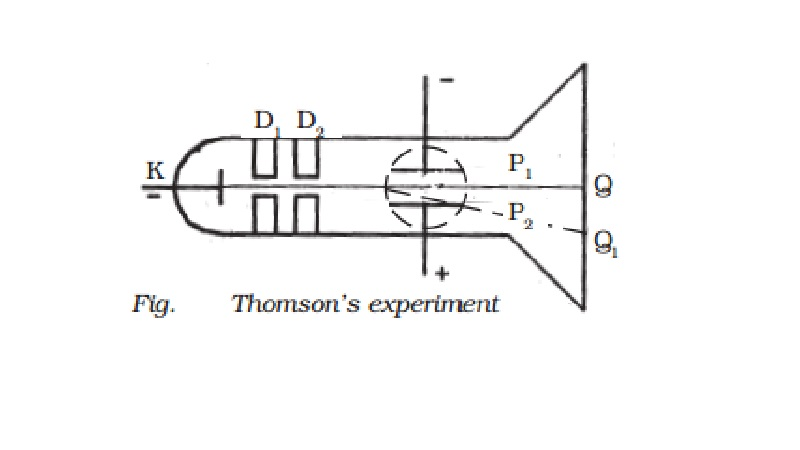Home | | Physics | | Physics | Thomson's method - Determination of specific charge (e/m) of an electron

# Thomson's method - Determination of specific charge (e/m) of an electronIn 1887, J.J. Thomson, measured the specific charge (e/m) of the cathode ray particles. The specific charge is defined as the charge per unit mass of the particle. Thomson discovered that the value of (e/m) was independent of the gas used and also independent of the nature of the electrodes.

Determination of specific charge (e/m) of an electron - Thomson's method.

In 1887, J.J. Thomson, measured the specific charge (e/m) of the cathode ray particles. The specific charge is defined as the charge per unit mass of the particle. Thomson discovered that the value of (e/m) was independent of the gas used and also independent of the nature of the electrodes.

Principle

The fact that the cathode rays (electrons) are deflected by electric and magnetic fields is made use of in this method.

Experimental arrangement

A highly evacuated discharge tube used in this experiment is as shown in Fig. Cathode rays are produced by the discharge between the cathode and the anodes D1 and D2. A thin pencil of cathode ray comes out through fine pin holes in the anode discs. The cathode    rays  then          pass between two parallel metal plates P1 and P2 and strike the flat face of the tube. This face is  coated with  suitable fluorescent material. A spot of light is produced at Q But when a potential difference V is applied between P1 and P2, the beam is deflected to point Q1. By the use of a pair of coils, uniform magnetic field is produced perpendicular to the plane of the paper and outwards through out the region between P1 P2.Theory

1. Determination of V

With a given electric intensity between the plates P1 and P2 the magnetic induction B is adjusted until the beam strikes the screen at the original position Q. Then the downward force Ee due to the electric field is balanced by the force Bev due to magnetic induction where e is the charge of the cathode ray particle and v is the velocity of the cathode rays.

Ee = Bev

V = E / B         ��. (1)

2. Determination of e/m

Now the magnetic induction is switched off. The deflection Q Q1 = y caused by the electric field alone is measured. At the instant when the cathode rays enter the region between the plates P1 and P2, initial velocity in the downward direction u = 0

Acceleration along the downward direction a = Ee/m , where m is the mass of each cathode ray particle.

Time for which the electron moves in the electric field t = l/v where l is the length of either of the plates and v is the velocity of the electron.

The deflection produced on the cathode rays along the downward direction in the electric field is given by

y1 = � (Ee/m) (l/v)2  �� (2)

Substituting for v from equation (1) in equation (2) we get

y1 = 1/2 (Ee/m)(l2/E2)B2 = �  ( el2B2 )  / (mE)

The displacement of the spot of light on the screen is given by (Fig)y = K y1, where K is a constant determined by the geometry of the discharge tube. Substituting for y1 we get,

y = K (1 e l2 B2)/2mE                ��.. (3)

e/m = 2yE / K l2 B2   ���.. (4)

By substituting the known values in the above relation e/m of an electron can be calculated. The value of e/m calculated using this experiment was found to be 1.7592 � 1011 C kg-1

Note: The value of e/m of an electron can also be calculated, by applying uniform magnetic field perpendicular to the path of the electron.

Study Material, Lecturing Notes, Assignment, Reference, Wiki description explanation, brief detail
11th 12th std standard Class Organic Inorganic Physical Chemistry Higher secondary school College Notes : Thomson's method - Determination of specific charge (e/m) of an electron |

Related Topics